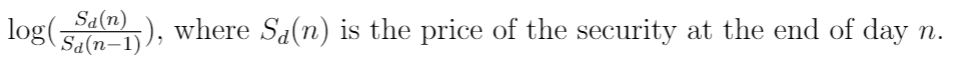(u)PSwhere Sa(n) is the price of the security at the end of daylogn.

Question

If the volatility of a stock is .33, find the standard deviation of:

(see the attachment for the remainder of the question).help_outlineImage Transcriptionclose(u)PS where Sa(n) is the price of the security at the end of day log n. fullscreen
Step 1

Recall the Black Scholes model derivation.

Log of the stock price is normally distributed.

The expected value of ln (ST / S0) is (µ - σ2 / 2) x T

Step 2

Hence, standard deviation of ln (ST / S0)  is σ x T1/2

Here, T = 1 day = 1/365

Hence, standard deviation of ln [Sd(n) / Sd(n...

Want to see the full answer?

See Solution

Want to see this answer and more?

Our solutions are written by experts, many with advanced degrees, and available 24/7

See Solution
Tagged in